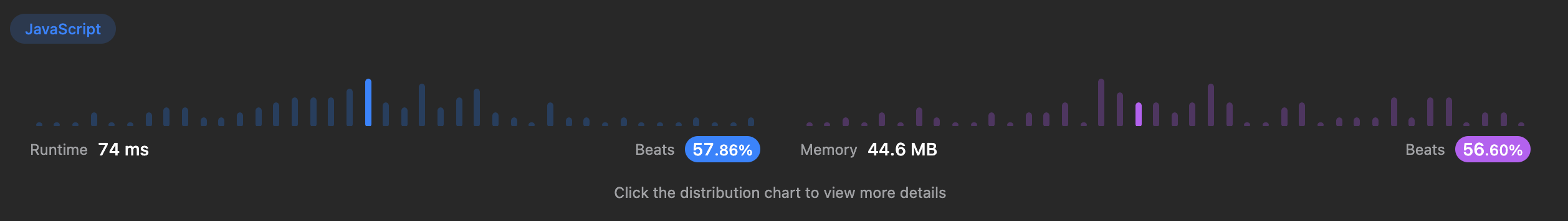# [LeetCode] Check Whether Two Strings are Almost Equivalent

jungyu333·2023년 3월 29일
0

## 1.문제

### Example 1

Input: word1 = "aaaa", word2 = "bccb"
Output: false
Explanation: There are 4 'a's in "aaaa" but 0 'a's in "bccb".
The difference is 4, which is more than the allowed 3.

### Example 2

Input: word1 = "abcdeef", word2 = "abaaacc"
Output: true
Explanation: The differences between the frequencies of each letter in word1 and word2 are at most 3:
- 'a' appears 1 time in word1 and 4 times in word2. The difference is 3.
- 'b' appears 1 time in word1 and 1 time in word2. The difference is 0.
- 'c' appears 1 time in word1 and 2 times in word2. The difference is 1.
- 'd' appears 1 time in word1 and 0 times in word2. The difference is 1.
- 'e' appears 2 times in word1 and 0 times in word2. The difference is 2.
- 'f' appears 1 time in word1 and 0 times in word2. The difference is 1.

### Example 3

Input: word1 = "cccddabba", word2 = "babababab"
Output: true
Explanation: The differences between the frequencies of each letter in word1 and word2 are at most 3:
- 'a' appears 2 times in word1 and 4 times in word2. The difference is 2.
- 'b' appears 2 times in word1 and 5 times in word2. The difference is 3.
- 'c' appears 3 times in word1 and 0 times in word2. The difference is 3.
- 'd' appears 2 times in word1 and 0 times in word2. The difference is 2.

### Constraints:

• n == word1.length == word2.length
• 1 <= n <= 100
• word1 and word2 consist only of lowercase English letters.

## 2.풀이

1. 두 문자열을 순회하면서 객체에 각 문자의 개수를 저장한다.
2. word1의 문자는 1씩 증가 , word2의 문자는 1씩 감소하게 한다.
3. 객체에서 각 value의 절대값이 3보다 작은지 체크한다.

/**
* @param {string} word1
* @param {string} word2
* @return {boolean}
*/
const checkAlmostEquivalent = function (word1, word2) {
let map = {};
for (let i = 0; i < word1.length; i++) {
// 두 배열을 순회하면서 word1의 문자의 개수는 1씩 증가 , word2의 문자는 1씩 감소 해서 객체에 저장한다
if (!map[word1[i]]) {
map[word1[i]] = 0;
}

if (!map[word2[i]]) {
map[word2[i]] = 0;
}

map[word1[i]]++;
map[word2[i]]--;
}

// 객체에서 value 값의 절댓값이 3보다 큰 경우가 있다면 false를 리턴한다
return !Object.keys(map).find((letter) => Math.abs(map[letter]) > 3);
};

## 3.결과